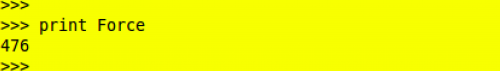HomeComputer programmingPython Programming: Introduction to Variables and Functions

# Python Programming: Introduction to Variables and Functions

#### Defining variables and functions in Python programming is a great way to simplify operations. In this tutorial, I will take you through this process by calculating the value of force for given values of mass and acceleration.

I will assume that you already have python installed and running. We will use the following common equation as an example in using functions and variables.

Force = Mass X Acceleration

1.The first step is to assign a value to each mass and acceleration (acce) using the equals sign (=). Remember to press the Return key after you finish typing each line. Each instance of mass or acceleration is known as variable and represents a memory location dedicated to holding the value. In this example, the value of the variable mass is 17 and that of acce is 28.2.By the way, the process of assigning a value to a variable as shown in Step 1 is called variable assignment. Still on variables, we can assign multiple variables on a single line using separating commas.3.In Step 3, we perform the multiplication operation using the asterisks sign (*). Typing Force = mass * acce and hitting the Return key does not return the expected value. This is because we have assigned the product of mass and acce to a new variable known as Force.4.In the next step, we print the value of Force. Note that the print command is required in order to access the value of a variable. Now, we have the desired value.5.We can sum up the steps above using in a function that we will call Force. Step 5 shows how a function is defined. The function is initialized with a def that is followed by the name of the new function we are defining (Force). In parenthesis are the parameters we are going to use to calculate the force. The line is then terminated with a colon (:). Pressing the Return key here takes you to the next line where you will need to enter one or two or more spaces before typing out the command. Once you are done, press the Return key twice to exit the function environment. You have just defined a new Python function.6.This step shows how a Python function is called by typing Function_name(parameters). Multiple parameters, like in this example, are separated with commas.7.Step 7 shows an important advantage of functions. A single function can be used for different parameters without the need to redefine new variables. To do this, we simply feed the new parameters into the function.Many lines of operation can be included during function definition. This makes it possible to perform very complex operations involving multiple steps by calling just one function.Alex Bahdanovich
Writer, author, wordsmith -- this tech enthusiast enjoys Starbucks, good reads, and golden retrievers.
RELATED ARTICLES